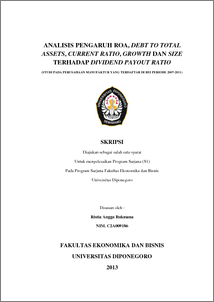# ANALISIS PENGARUH ROA, DEBT TO TOTAL ASSETS, CURRENT RATIO, GROWTH DAN SIZE TERHADAP DIVIDEND PAYOUT RATIO (STUDI PADA PERUSAHAAN MANUFAKTUR YANG TERDAFTAR DI BEI PERIODE 2007-2011)

RUKMANA, Ristia Angga and WIDYARTI, Endang Tri (2013) ANALISIS PENGARUH ROA, DEBT TO TOTAL ASSETS, CURRENT RATIO, GROWTH DAN SIZE TERHADAP DIVIDEND PAYOUT RATIO (STUDI PADA PERUSAHAAN MANUFAKTUR YANG TERDAFTAR DI BEI PERIODE 2007-2011). Undergraduate thesis, Fakultas Ekonomika dan Bisnis.Preview
PDF - Published Version
23Mb

## Abstract

This study conducted to analyze the effect of variable Return On Assets (ROA), Debt To Total Assets (DTA), Current Ratio, Growth and Size to the Dividend Payout Ratio oncompany listed on the Jakarta Stock Exchangefrom 2007 – 2011. The sampling technique used was purposive sampling and number of samples used by 17 companies. Variables used in this study were, Dividend Payout Ratio (DPR) as the dependent variable and the Return On Assets (ROA), Current Ratio, Growth, and Size as an independent variable. Data obtained from the Indonesian Capital Market Directory (ICMD 2007-2011) and the financial statements of the company (2007-2011). The analysis technique used is multiple linear regression and hypothesis testing using the F statistic to test the effect together with a 5% confidence level and t-statistics for testing the partial regression coefficients. The results showed that the variables ROA and Debt to total assets has positive and significant impact to the DPR. While the variable Current Ratio, Growth and Size positive and significant effect to the DPR. From 5 hypothesis, there is only one hypothesis are accepted, ROA has positive and significant impact. Based on results of statistical analysis F, obtained significant value 0.010 (less than 0.05) and it can be concluded that the variables ROA, Debt to total assets, Current ratio, Growth, and Size have a significant effect to the DPR. Of the value of determination coefficient of 0.191 indicates that the model can explain the variation (rise and fall) DPR of 19,1% while 80.1% the variation is explained by other variables outside the model

Item Type: Thesis (Undergraduate) Dividend Payout Ratio, Return on assets, Debt to total assets, Current Ratio, Growth, Size Dividend Payout Ratio, Return on assets, Debt to total assets, Current Ratio, Growth, Size H Social Sciences > H Social Sciences (General) Faculty of Economics and Business > Department of Management 39694 Mr. Perpustakaan Fakultas Ekonomi 01 Aug 2013 08:49 08 May 2014 09:54

Repository Staff Only: item control page